# Assignments

The following assignments relate to all kinds of basic skills. Some parts are dealt with separately in the other topics.

1. Simplify: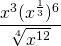Solution

2. Factorize: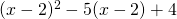Solution

3. Factorize: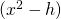als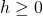Solution

4. Calculate the value of the following fraction as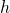approaches to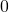: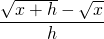Note that if you just substitutethe result iswhich is not defined. The solution of this assignment is used in determining the derivative of the function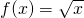, see also the subject Differentiating basic functions.

Solution

5. Calculate without the aid of a calculator: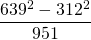Solution

6. Solve the following equation: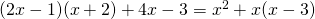Solution

7. Solve the following equations with the sum-product method: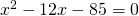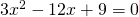Solution

8. Solve the following equations: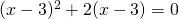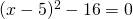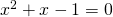Solution

9. Factorize: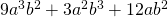Solution

10. Calculate without the aid of a calculator:.

Solution

0